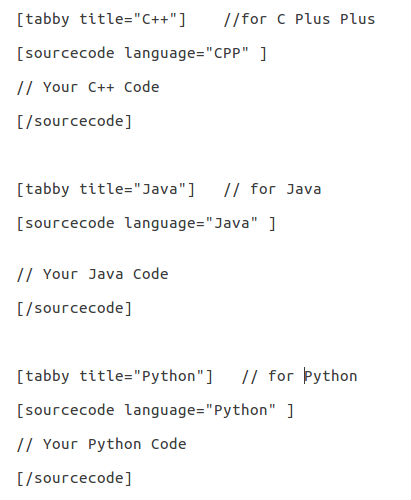# Guidelines for adding C/C++/Java/Python code to existing articles of GeeksforGeeks

You may please first go through code writing instructions: Guide to write an article

Guidelines for writing code for an existing code on GeeksforGeeks:

1. This guideline is for those who directly write in http://contribute.geeksforgeeks.org/. To write code in multiple languages use the format shown below:2. Please note that all of these attributes must match in all the programs:
1. Variable names
2. Function names
4. Output
5. Approach used (Say, if stack is used to solve a problem, then all problems MUST be solved using stack)

See the following example:

## C

```// A Sample C program for beginners with Competitive Programming
#include<stdio.h>

// This function returns index of element x in arr[]
int search(int arr[], int n, int x)
{
int i;
for (i = 0; i < n; i++)
{
// Return the index of the element if the element
// is found
if (arr[i] == x)
return i;
}

return -1;
}

int main()
{
// Note that size of arr[] is considered 100 according to
// the constraints mentioned in problem statement.
int arr, x, t, n, i;

// Input the number of test cases you want to run
scanf("%d", &t);

// One by one run for all input test cases
while (t--)
{
// Input the size of the array
scanf("%d", &n);

// Input the array
for (i=0; i<n; i++)
scanf("%d",&arr[i]);

// Input the element to be searched
scanf("%d", &x);

// Compute and print result
printf("%d\n", search(arr, n, x));
}
return 0;
}
```

## C++

```// A Sample C++ program for beginners with Competitive Programming
#include<iostream>
using namespace std;

// This function returns index of element x in arr[]
int search(int arr[], int n, int x)
{
for (int i = 0; i < n; i++)
{
// Return the index of the element if the element
// is found
if (arr[i] == x)
return i;
}

return -1;
}

int main()
{
// Note that size of arr[] is considered 100 according to
// the constraints mentioned in problem statement.
int arr, x, t, n;

// Input the number of test cases you want to run
cin >> t;

// One by one run for all input test cases
while (t--)
{
// Input the size of the array
cin >> n;

// Input the array
for (int i=0; i<n; i++)
cin >> arr[i];

// Input the element to be searched
cin >> x;

// Compute and print result
cout << search(arr, n, x) << endl;
}
return 0;
}
```

## Python

```# A Sample Python program for beginners with Competitive Programming

# Returns index of x in arr if it is present,
# else returns -1
def search(arr, x):
n = len(arr)
for j in range(0,n):
if (x == arr[j]):
return j
return -1

# Input number of test cases
t = int(raw_input())

# One by one run for all input test cases
for i in range(0,t):

# Input the size of the array
n = int(raw_input())

# Input the array
arr = map(int, raw_input().split())

# Input the element to be searched
x = int(raw_input())

print(search(arr, x))

# The element can also be searched by index method
# But you need to handle the exception when element is not found
# Uncomment the below line to get that working.
# arr.index(x)
```

## Java

```// A Sample Java program for beginners with Competitive Programming
import java.util.*;
import java.lang.*;
import java.io.*;

class LinearSearch
{
// This function returns index of element x in arr[]
static int search(int arr[], int n, int x)
{
for (int i = 0; i < n; i++)
{
// Return the index of the element if the element
// is found
if (arr[i] == x)
return i;
}

return -1;
}

public static void main (String[] args)
{
// Note that size of arr[] is considered 100 according to
// the constraints mentioned in problem statement.
int[] arr = new int;

// Input the number of test cases you want to run
Scanner sc = new Scanner(System.in);
int t = sc.nextInt();

// One by one run for all input test cases
while (t > 0)
{
// Input the size of the array
int n = sc.nextInt();

// Input the array
for (int i = 0; i < n; i++)
arr[i] = sc.nextInt();

// Input the element to be searched
int x = sc.nextInt();

// Compute and print result
System.out.println(search(arr, n, x));

t--;
}
}
}
```

` // This code is contributed by Amit Khandelwal`